SBAS825 April   2017

PRODUCTION DATA.

1. Features
2. Applications
3. Description
4. Revision History
5. Device Family Comparison Table
6. Pin Configuration and Functions
7. Specifications
8. Detailed Description
1. 8.1 Overview
2. 8.2 Functional Block Diagram
3. 8.3 Feature Description
4. 8.4 Device Functional Modes
1. 8.4.1 Device Interface: Pin Description
2. 8.4.2 Device Modes of Operation
1. 8.4.2.1 Power-Down Modes
2. 8.4.2.2 Conversion Control
4. 8.4.2.4 Oversampling Mode of Operation
9. Application and Implementation
1. 9.1 Application Information
2. 9.2 Typical Application
10. 10Power Supply Recommendations
11. 11Layout
12. 12Device and Documentation Support
13. 13Mechanical, Packaging, and Orderable Information

• PM|64

## 7 Specifications

### 7.1 Absolute Maximum Ratings

at TA = 25°C (unless otherwise noted)(1)
MIN MAX UNIT
AVDD to AGND –0.3 7.0 V
DVDD to AGND –0.3 7.0 V
Digital input to AGND –0.3 DVDD + 0.3 V
REFIN to AGND –0.3 AVDD + 0.3 V
Input current to any pin except supplies(2) –10 10 mA
Temperature Operating –40 125 °C
Junction, TJ 150
Storage, Tstg –65 150
Stresses beyond those listed under Absolute Maximum Ratings may cause permanent damage to the device. These are stress ratings only, which do not imply functional operation of the device at these or any other conditions beyond those indicated under Recommended Operating Conditions. Exposure to absolute-maximum-rated conditions for extended periods may affect device reliability.
Transient currents of up to 100 mA do not cause SCR latch-up.

### 7.2 ESD Ratings

VALUE UNIT
V(ESD) Electrostatic discharge Human-body model (HBM), per ANSI/ESDA/JEDEC JS-001(1) All pins except analog inputs ±2000 V
Charged-device model (CDM), per JEDEC specification JESD22-C101(2) All pins ±500
JEDEC document JEP155 states that 500-V HBM allows safe manufacturing with a standard ESD control process.
JEDEC document JEP157 states that 250-V CDM allows safe manufacturing with a standard ESD control process.

### 7.3 Recommended Operating Conditions

over operating free-air temperature range (unless otherwise noted)
MIN NOM MAX UNIT
AVDD Analog supply voltage 4.75 5 5.25 V
DVDD Digital supply voltage 2.3 3.3 AVDD V

### 7.4 Thermal Information

PM (LQFP)
64 PINS
RθJA Junction-to-ambient thermal resistance 46.0 °C/W
RθJC(top) Junction-to-case (top) thermal resistance 7.8 °C/W
RθJB Junction-to-board thermal resistance 20.1 °C/W
ψJT Junction-to-top characterization parameter 0.3 °C/W
ψJB Junction-to-board characterization parameter 19.6 °C/W
RθJC(bot) Junction-to-case (bottom) thermal resistance N/A °C/W

### 7.5 Electrical Characteristics

minimum and maximum specifications are at TA = –40°C to +125°C, AVDD = 4.75 V to 5.25 V; typical specifications are at TA = 25°C; AVDD = 5 V, DVDD = 3 V, VREF = 2.5 V (internal), and fSAMPLE = 200 kSPS (unless otherwise noted)
PARAMETER TEST CONDITIONS MIN TYP MAX UNIT TEST LEVEL(8)
Full-scale input span(1)
(AIN_nP to AIN_nGND)
RANGE pin = 1 –10 10 V A
RANGE pin = 0 –5 5 A
AIN_nP Operating input range,
positive input
RANGE pin = 1 –10 10 V A
RANGE pin = 0 –5 5 A
AIN_nGND Operating input range,
negative input
All input ranges –0.3 0 0.3 V B
RIN Input impedance At TA = 25°C 0.85 1 1.15 B
Input impedance drift All input ranges –25 ±7 25 ppm/°C B
IIkg(in) Input leakage current With voltage at AIN_nP = VIN,
all input ranges
(VIN – 2) / RIN µA A
SYSTEM PERFORMANCE
Resolution 14 Bits A
NMC No missing codes 14 Bits A
DNL Differential nonlinearity All input ranges –0.5 ±0.2 0.5 LSB(2) A
INL Integral nonlinearity(4) All input ranges –0.45 ±0.2 0.45 LSB A
EG Gain error(9) All input ranges,
external reference
TA = –40°C to +85°C –16 ±1 16 LSB A
TA = –40°C to +125°C –16 ±1 24 A
All input ranges,
internal reference
±0.3 A
Gain error matching
(channel-to-channel)
Input range = ±10 V,
external and internal reference
2 15 LSB A
Input range = ±5 V,
external and internal reference
2.5 15 A
Gain error temperature drift All input ranges,
external reference
–14 ±6 14 ppm/°C B
All input ranges,
internal reference
±10 B
EO Offset error Input range = ±10 V –1.8 ±0.15 1.8 mV B
Input range = ±5 V –1.8 ±0.15 1.8 B
Offset error matching
(channel-to-channel)
All input ranges 0.3 2.4 mV B
Offset error temperature drift All input ranges –3 ±0.3 3 ppm/°C B
SAMPLING DYNAMICS
tACQ Acquisition time 1 µs A
fS Maximum throughput rate per channel without latency All eight channels included 200 kSPS A
DYNAMIC CHARACTERISTICS
SNR Signal-to-noise ratio,
no oversampling
(VIN – 0.5 dBFS at 1 kHz)
Input range = ±10 V 84.5 85.7 dB A
Input range = ±5 V 84.2 85.6 A
SNROSR Signal-to-noise ratio,
oversampling = 4x
(VIN – 0.5 dBFS at 130 Hz)
Input range = ±10 V 85.5 85.8 dB A
Input range = ±5 V 85.2 85.6 A
THD Total harmonic distortion(3)
(VIN – 0.5 dBFS at 1 kHz)
All input ranges –109 –95 dB B
no oversampling
(VIN – 0.5 dBFS at 1 kHz)
Input range = ±10 V 84.5 85.4 dB A
Input range = ±5 V 84.3 85.3 A
oversampling = 4x
(VIN – 0.5 dBFS at 130 Hz)
Input range = ±10 V 85.4 85.5 dB A
Input range = ±5 V 85.2 85.4 A
SFDR Spurious-free dynamic range
(VIN – 0.5 dBFS at 1 kHz)
All input ranges –111 dB B
Crosstalk isolation(5) –95 dB A
BW(–3 dB) Small-signal bandwidth –3 dB At TA = 25°C,
input range = ±10 V
24 kHz B
At TA = 25°C,
input range = ±5 V
16 B
BW(–0.1 dB) –0.1 dB At TA = 25°C,
input range = ±10 V
14 kHz B
At TA = 25°C,
input range = ±5 V
9.5 B
tGROUP Group delay Input range = ±10 V 13 µs C
Input range = ±5 V 19 C
INTERNAL REFERENCE OUTPUT (REFSEL = 1)
VREF(6) Voltage on the REFIN/REFOUT pin
(configured as output)
At TA = 25°C 2.4975 2.5 2.5025 V A
Internal reference temperature drift 7.5 ppm/°C B
C(REFIN_ REFOUT) Decoupling capacitor on REFIN/REFOUT(7) 10 µF B
V(REFCAP) Reference voltage to the ADC
(on the REFCAPA, REFCAPB pin)
At TA = 25°C 3.996 4.0 4.004 V A
Reference buffer output impedance 0.5 1 Ω C
Reference buffer output temperature drift 5 ppm/°C B
C(REFCAP) Decoupling capacitor on REFCAPA, REFCAPB 10 μF B
Turn-on time C(REFCAP) = 10 µF,
C(REFIN_REFOUT) = 10 µF
25 ms B
EXTERNAL REFERENCE INPUT (REFSEL = 0)
VREFIO_EXT External reference voltage on REFIO
(configured as input)
2.475 2.5 2.525 V B
Reference input impedance 100 C
Reference input capacitance 10 pF C
POWER-SUPPLY REQUIREMENTS
AVDD Analog power-supply voltage Analog supply 4.75 5 5.25 V A
DVDD Digital power-supply voltage Digital supply range 2.3 3.3 AVDD V A
IAVDD_DYN Analog supply current
(operational)
For ADS8578S, AVDD = 5 V,
fS = 200 kSPS,
internal reference
17.7 24.0 mA A
For ADS8578S, AVDD = 5 V,
fS = 200 kSPS,
external reference
17.1 24.0 A
IAVDD_STC Analog supply current
(static)
For ADS8578S, AVDD = 5 V, internal reference,
device not converting
12.4 17.0 mA A
For ADS8578S, AVDD = 5 V, external reference,
device not converting
12.0 17.0 A
IAVDD_STDBY AVDD supply
STANDBY current
At AVDD = 5 V, device in STDBY mode, internal reference 4.2 5.5 mA A
At AVDD = 5 V, device in STDBY mode, external reference 3.8 5.5 A
IAVDD_PWR_ DN AVDD supply
power-down current
At AVDD = 5 V, device in PWR_DN, internal or external reference,
TA = –40°C to +85°C
0.2 6 µA A
IDVDD_DYN Digital supply current For ADS8578S,
DVDD = 3.3 V,
fS = 200 kSPS
0.15 0.3 mA A
IDVDD_STDBY DVDD supply STANDBY current At AVDD = 5 V, device in STDBY mode 0.05 1.5 µA A
IDVDD_PWR-DN DVDD supply power-down current At AVDD = 5 V, device in PWR_DN mode 0.05 1.5 µA A
DIGITAL INPUTS (CMOS)
VIH Digital high input voltage logic level DVDD > 2.3 V 0.7 × DVDD DVDD + 0.3 V A
VIL Digital low input voltage logic level DVDD > 2.3 V –0.3 0.3 × DVDD V A
Input leakage current 100 nA A
Input pin capacitance 5 pF A
DIGITAL OUTPUTS (CMOS)
VOH Digital high output voltage logic level IO = 100-μA source 0.8 × DVDD DVDD V A
VOL Digital low output voltage logic level IO = 100-μA sink 0 0.2 × DVDD V A
Floating state leakage current Only for SDO 1 µA A
Internal pin capacitance 5 pF A
TEMPERATURE RANGE
TA Operating free-air temperature –40 125 °C A
Ideal input span, does not include gain or offset error.
LSB = least significant bit.
Calculated on the first nine harmonics of the input frequency.
This parameter is the endpoint INL, not best-fit INL.
Isolation crosstalk is measured by applying a full-scale sinusoidal signal up to 160 kHz to a channel, not selected in the multiplexing sequence, and measuring the effect on the output of any selected channel.
Does not include the variation in voltage resulting from solder shift effects.
Recommended to use an X7R-grade, 0603-size ceramic capacitor for optimum performance (see the Layout Guidelines section).
Test Levels: (A) Tested at final test. Overtemperature limits are set by characterization and simulation. (B) Limits set by characterization and simulation, across temperature range. (C) Typical value only for information, provided by design simulation.
Gain error is calculated after adjusting for offset error, which implies that positive full scale error = negative full scale error = gain error ÷ 2.

### 7.6 Timing Requirements: CONVST Control

minimum and maximum specifications are at TA = –40°C to +125°C, typical specifications are at TA = 25°C; AVDD = 5 V, 2.3 V ≤ DVDD ≤ 5.25 V, VREF = 2.5 V (internal), BUSY load = 20 pF, VIL and VIH at datasheet limits, and fSAMPLE = 200 kSPS (unless otherwise noted) (see Figure 1)
MIN NOM MAX UNIT
tACQ Acquisition time:
BUSY falling edge to rising edge of trailing CONVSTA or CONVSTB
1 µs
tPH_CN CONVSTA, CONVSTB pulse high time 25 ns
tPL_CN CONVSTA, CONVSTB pulse low time 25 ns
tSU_BSYCS Setup time: BUSY falling to CS falling 0 ns
tSU_RSTCN Setup time: RESET falling to first rising edge of CONVSTA or CONVSTB 25 ns
tPH_RST RESET pulse high time 50 ns
tD_CNAB Delay between rising edges of CONVSTA and CONVSTB 500 µs

### 7.7 Timing Requirements: Data Read Operation

minimum and maximum specifications are at TA = –40°C to +125°C, typical specifications are at TA = 25°C; AVDD = 5 V, 2.3 V ≤ DVDD ≤ 5.25 V, VREF = 2.5 V (internal), BUSY load = 20 pF, VIL and VIH at datasheet limits, and fSAMPLE = 200 kSPS (unless otherwise noted) (see Figure 2)
MIN NOM MAX UNIT
tDZ_CNCS Delay between CONVSTA, CONVSTB rising edge to CS falling edge, start of data read operation during conversion 10 ns
tDZ_CSBSY Delay between CS rising edge to BUSY falling edge, end of data read operation during conversion 40 ns
tSU_BSYCS Setup time: BUSY falling edge to CS falling edge, start of data read operation after conversion 0 ns
tD_CSCN Delay between CS rising edge to CONVSTA, CONVSTB rising edge, end of data read operation after conversion 10 ns

### 7.8 Timing Requirements: Parallel Data Read Operation, CS and RD Tied Together

minimum and maximum specifications are at TA = –40°C to +125°C, typical specifications are at TA = 25°C; AVDD = 5 V, 2.3 V ≤ DVDD ≤ 5.25 V, VREF = 2.5 V (internal), load on DB[15:0] and FRSTDATA = 20 pF, VIL and VIH at datasheet limits, and fSAMPLE = 200 kSPS (unless otherwise noted) (see Figure 3)
MIN NOM MAX UNIT
tPH_CS, tPH_RD CS and RD high time 15 ns
tPL_CS, tPL_RD CS and RD low time 15 ns
tHT_RDDB, tHT_CSDB Hold time: RD and CS rising edge to DB[15:0] invalid 2.5 ns

### 7.9 Timing Requirements: Parallel Data Read Operation, CS and RD Separate

minimum and maximum specifications are at TA = –40°C to +125°C, typical specifications are at TA = 25°C; AVDD = 5 V, 2.3 V ≤ DVDD ≤ 5.25 V, VREF = 2.5 V (internal), load on DB[15:0] and FRSTDATA = 20 pF, VIL and VIH at datasheet limits, and fSAMPLE = 200 kSPS (unless otherwise noted) (see Figure 4)
MIN NOM MAX UNIT
tSU_CSRD Set-up time: CS falling edge to RD falling edge 0 ns
tHT_RDCS Hold time: RD rising edge to CS rising edge 0 ns
tPL_RD RD low time 15 ns
tPH_RD RD high time 15 ns
tHT_CSDB Hold time: CS rising edge to DB[15:0] becoming invalid 6 ns
tHT_RDDB Hold time: RD rising edge to DB[15:0] becoming invalid 2.5 ns

### 7.10 Timing Requirements: Serial Data Read Operation

minimum and maximum specifications are at TA = –40°C to +125°C, typical specifications are at TA = 25°C; AVDD = 5 V, 2.3 V ≤ DVDD ≤ 5.25 V, VREF = 2.5 V (internal), load on DOUTA, DOUTB, and FRSTDATA = 20 pF, VIL and VIH at datasheet limits, and fSAMPLE = 200 kSPS (unless otherwise noted) (see Figure 5)
MIN NOM MAX UNIT
tSCLK SCLK time period 50 ns
tPH_SCLK SCLK high time 0.45 0.55 tSCLK
tPL_SCLK SCLK low time 0.45 0.55 tSCLK
tHT_CKDO Hold time: SCLK rising edge to DOUTA, DOUTB invalid 7 ns
tSU_CSCK Setup time: CS falling to first SCLK edge 8 ns
tHT_CKCS Hold time: last SCLK active edge to CS high 10 ns

### 7.11 Timing Requirements: Byte Mode Data Read Operation

minimum and maximum specifications are at TA = –40°C to +125°C, typical specifications are at TA = 25°C; AVDD = 5 V, 2.3 V ≤ DVDD ≤ 5.25 V, VREF = 2.5 V (internal), load on DB[7:0] and FRSTDATA = 20 pF, VIL and VIH at datasheet limits, and fSAMPLE = 200 kSPS (unless otherwise noted) (see Figure 6)
MIN NOM MAX UNIT
tSU_CSRD Setup time: CS falling edge to RD falling edge 0 ns
tHT_RDCS Hold time: RD rising edge to CS rising edge 0 ns
tPL_RD RD low time 15 ns
tPH_RD RD high time 15 ns
tHT_CSDB Hold time: CS rising edge to DB[15:0] becoming invalid 6 ns
tHT_RDDB Hold time: RD rising edge to DB[15:0] becoming invalid 2.5 ns

### 7.12 Timing Requirements: Oversampling Mode

MIN NOM MAX UNIT
tHT_OS Hold time: BUSY falling to OSx 20 ns
tSU_OS Setup time: BUSY falling to OSx 20 ns

### 7.13 Timing Requirements: Exit Standby Mode

minimum and maximum specifications are at TA = –40°C to +125°C, typical specifications are at TA = 25°C, AVDD = 5 V, 2.3 V ≤ DVDD ≤ 5.25 V, VREF = 2.5 V (internal), VIL and VIH at datasheet limits, and fSAMPLE = 200 kSPS (unless otherwise noted) (see Figure 8)
MIN NOM MAX UNIT
tD_STBYCN Delay between STBY rising edge to CONVSTA or CONVSTB rising edge(1) 100 µs
First conversion data must be discarded or RESET must be issued if the maximum timing is exceeded.

### 7.14 Timing Requirements: Exit Shutdown Mode

minimum and maximum specifications are at TA = –40°C to +125°C, typical specifications are at TA = 25°C; AVDD = 5 V, 2.3 V ≤ DVDD ≤ 5.25 V, VREF = 2.5 V (internal), VIL and VIH at datasheet limits, and fSAMPLE = 200 kSPS (unless otherwise noted) (see Figure 9)
MIN NOM MAX UNIT
tD_SDRST Delay between STBY rising edge to RESET rising edge Internal reference mode 50 ms
External reference mode(1) 13
tPH_RST RESET high time 50 ns
tD_RSTCN Delay between RESET falling edge to CONVSTA or CONVSTB rising edge 25 µs
Excludes wake-up time for external reference device.

### 7.15 Switching Characteristics: CONVST Control

minimum and maximum specifications are at TA = –40°C to +125°C, typical specifications are at TA = 25°C; AVDD = 5 V, 2.3 V ≤ DVDD ≤ 5.25 V, VREF = 2.5 V (internal), BUSY load = 20 pF, VIL and VIH at datasheet limits, and fSAMPLE = 200 kSPS (unless otherwise noted) (see Figure 1)
PARAMETER TEST CONDITIONS MIN TYP MAX UNIT
tCYC ADC cycle time period No oversampling, parallel read, serial read with both DOUTA and DOUTB during conversion 5 µs
No oversampling, serial read after conversion with both DOUTA and DOUTB, 7
No oversampling, serial read after conversion with only DOUTA or DOUTB, 9.7
tCONV Conversion time: BUSY high time No oversampling, 8 channels 3.7 3.8 3.9 µs
No oversampling, 6 channels 3
No oversampling, 4 channels 2
Oversampling by 2 8.4 8.8
Oversampling by 4 17.5 18.5
Oversampling by 8 36 38
Oversampling by 16 73 77
Oversampling by 32 148 155
Oversampling by 64 298 311
tD_CNBSY Delay between trailing rising edges of CONVSTA or CONVSTB and BUSY rising 15 ns

### 7.16 Switching Characteristics: Parallel Data Read Operation, CS and RD Tied Together

minimum and maximum specifications are at TA = –40°C to +125°C, typical specifications are at TA = 25°C; AVDD = 5 V, 2.3 V ≤ DVDD ≤ 5.25 V, VREF = 2.5 V (internal), load on DB[15:0] and FRSTDATA = 20 pF, VIL and VIH at datasheet limits, and fSAMPLE = 200 kSPS (unless otherwise noted) (see Figure 3)
PARAMETER TEST CONDITIONS MIN TYP MAX UNIT
tD_CSDB Delay time: CS falling edge to DB[15:0] becoming valid
(out of tri-state)
12 ns
tD_RDDB Delay time: RD falling edge to new data on DB[15:0] 17 ns
tD_CSFD, tD_RDFD Delay time: CS and RD falling edge to FRSTDATA going high or low out of tri-state 10 ns
tDHZ_CSDB, tDHZ_RDDB Delay time: CS and RD rising edge to DB[15:0] tri-state 12 ns
tDHZ_CSFD, tDHZ_RDFD Delay time: CS and RD rising edge to FRSTDATA tri-state 10 ns

### 7.17 Switching Characteristics: Parallel Data Read Operation, CS and RD Separate

minimum and maximum specifications are at TA = –40°C to +125°C, typical specifications are at TA = 25°C; AVDD = 5 V, 2.3 V ≤ DVDD ≤ 5.25 V, VREF = 2.5 V (internal), load on DB[15:0] and FRSTDATA = 20 pF, VIL and VIH at datasheet limits, and fSAMPLE = 200 kSPS (unless otherwise noted) (see Figure 4)
PARAMETER TEST CONDITIONS MIN TYP MAX UNIT
tD_CSDB Delay time: CS falling edge to DB[15:0] becoming valid
(out of tri-state)
12 ns
tD_RDDB Delay time: RD falling edge to new data on DB[15:0] 17 ns
tDHZ_CSDB Delay time: CS rising edge to DB[15:0] becoming tri-state 12 ns
tD_CSFD Delay time: CS falling edge to FRSTDATA going low out of tri-state 15 ns
tDHZ_CSFD Delay time: CS rising edge to FRSTDATA going to tri-state 10 ns
tD_RDFD Delay time: RD falling edge to FRSTDATA going high or low 15 ns

### 7.18 Switching Characteristics: Serial Data Read Operation

minimum and maximum specifications are at TA = –40°C to +125°C, typical specifications are at TA = 25°C; AVDD = 5 V, 2.3 V ≤ DVDD ≤ 5.25 V, VREF = 2.5 V (internal), load on DOUTA, DOUTB, and FRSTDATA = 20 pF, VIL and VIH at datasheet limits, and fSAMPLE = 200 kSPS (unless otherwise noted) (see Figure 5)
PARAMETER TEST CONDITIONS MIN TYP MAX UNIT
tD_CSDO Delay time: CS falling edge to DOUTA, DOUTB enable
(out of tri-state)
12 ns
tD_CKDO Delay time: SCLK rising edge to valid data on DOUTA, DOUTB 15 ns
tDZ_CSDO Delay time: CS rising edge to DOUTA, DOUTB going to tri-state 12 ns
tD_CSFD Delay time: CS falling edge to FRSTDATA from tri-state to high or low 10 ns
tDZ_CKFD Delay time: 14th SCLK falling edge to FRSTDATA falling edge 15 ns
tDHZ_CSFD Delay time: CS rising edge to FRSTDATA going to tri-state 10 ns

### 7.19 Switching Characteristics: Byte Mode Data Read Operation

minimum and maximum specifications are at TA = –40°C to +125°C, typical specifications are at TA = 25°C; AVDD = 5 V, 2.3 V ≤ DVDD ≤ 5.25 V, VREF = 2.5 V (internal), load on DB[7:0] and FRSTDATA = 20 pF, VIL and VIH at datasheet limits, and fSAMPLE = 200 kSPS (unless otherwise noted) (see Figure 6)
PARAMETER TEST CONDITIONS MIN TYP MAX UNIT
tD_CSDB Delay time: CS falling edge to DB[7:0] becoming valid
(out of tri-state)
12 ns
tD_RDDB Delay time: RD falling edge to new data on DB[7:0] 17 ns
tDHZ_CSDB Delay time: CS rising edge to DB[7:0] becoming tri-state 12 ns
tD_CSFD Delay time: CS falling edge to FRSTDATA going low out of tri-state 10 ns
tD_RDFD Delay time: RD falling edge to FRSTDATA going low or high state 15 ns
tDHZ_CSFD Delay time: CS rising edge to FRSTDATA going to tri-state 10 ns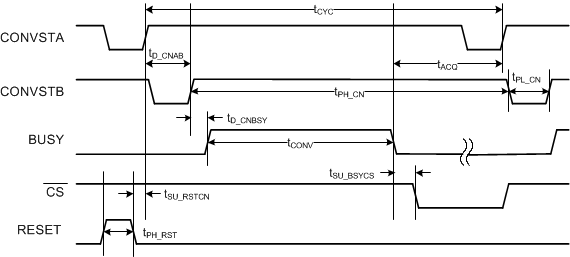Figure 1. CONVST Control Timing DiagramFigure 2. Data Read Operation Timing Diagram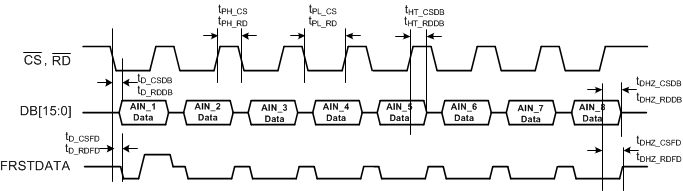Figure 3. Parallel Data Read Operation, CS and RD Tied Together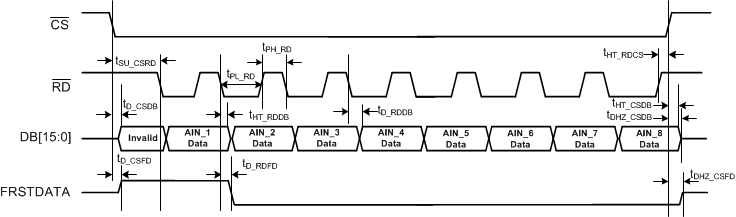Figure 4. Parallel Data Read Operation, CS and RD Separate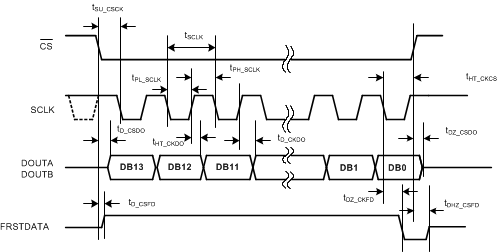Figure 5. Serial Data Read Operation Timing Diagram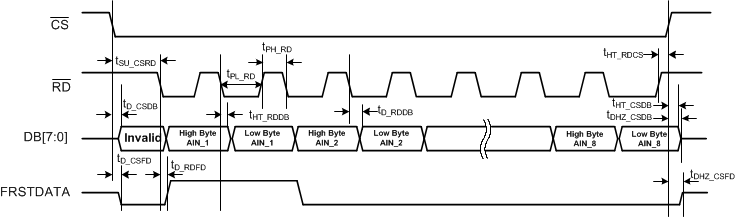Figure 6. Byte Mode Data Read Operation Timing Diagram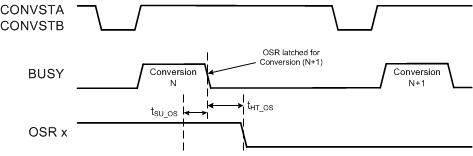Figure 7. Oversampling Mode Timing Diagram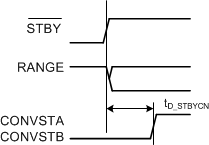Figure 8. Exit Standby Mode Timing Diagram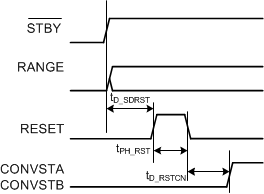Figure 9. Exit Shutdown Mode Timing Diagram

### 7.20 Typical Characteristics

at TA = 25°C, AVDD = 5 V, DVDD = 3 V, internal reference VREF = 2.5 V, and fS = 200 kSPS per channel (unless otherwise noted)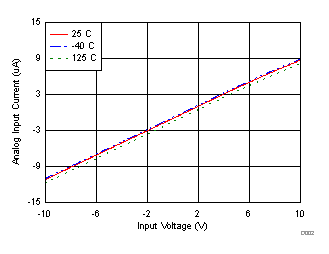Figure 10. Analog Input Current vs Input Voltage Over Temperature (±10 V)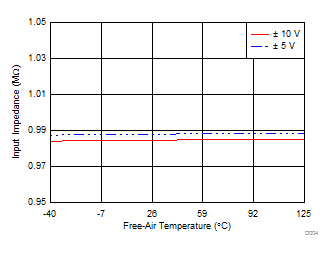Figure 12. Input Impedance vs Temperature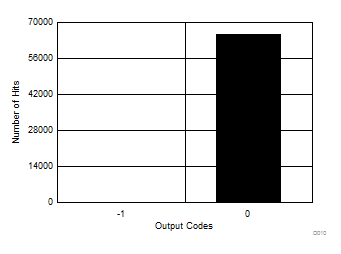Mean = –0.001, sigma = 0.04, number of hits = 65536, VIN = 0 V
Figure 14. DC Histogram of Codes (±5 V)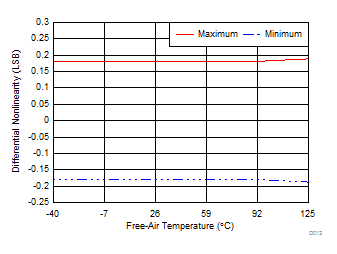Figure 16. DNL vs Temperature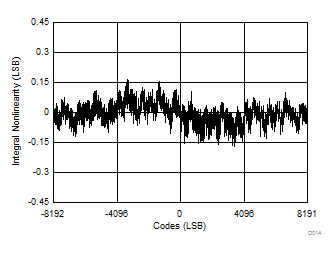Figure 18. INL vs All Codes (±5 V)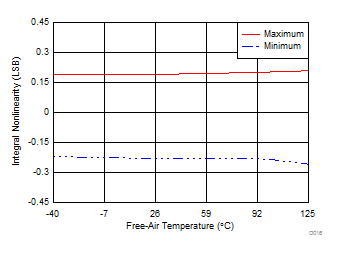Figure 20. INL vs Temperature (±5 V)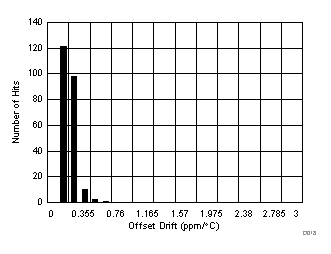Figure 22. Offset Drift Histogram Distribution (±10 V)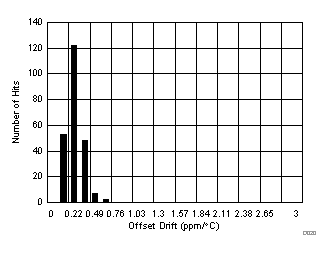Figure 24. Offset Drift Histogram Distribution (±5 V)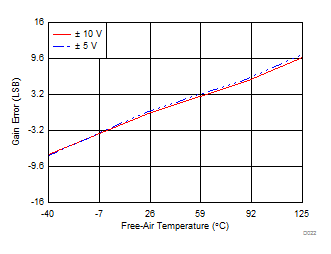External reference
Figure 26. Gain Error vs Temperature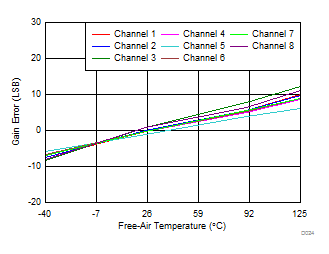External reference
Figure 28. Gain Error Across Channels vs Temperature
(±10 V)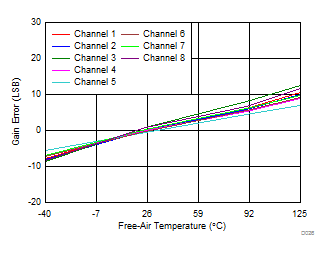External reference
Figure 30. Gain Error Across Channels vs Temperature
(±5 V)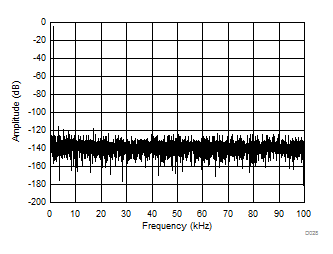Number of points = 32k, SNR = 85.41 dB, SINAD = 85.38 dB, THD = –110.57 dB, SFDR = 114.17 dB
Figure 32. Typical FFT Plot (±10 V)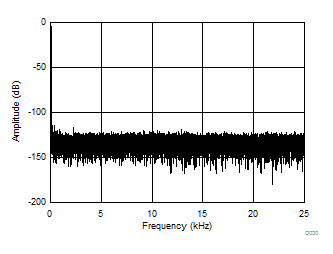Number of points = 32k, SNR = 85.66 dB, SINAD = 85.62 dB, THD = –110.63 dB, SFDR = 114.65 dB
Figure 34. Typical FFT Plot for OSR 4x (±10 V)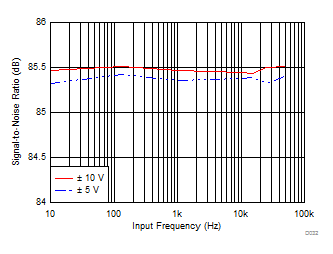OSR = 0
Figure 36. SNR vs Input Frequency for Different Input Ranges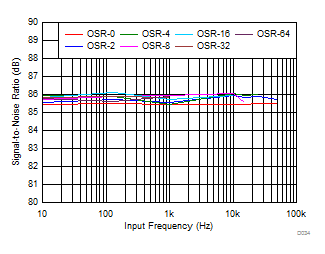Figure 38. SNR vs Input Frequency for Different OSR
(±10 V)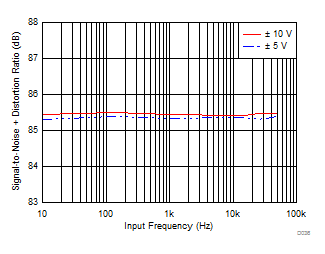OSR = 0
Figure 40. SINAD vs Input Frequency for Different Input RangesFigure 42. THD vs Input Frequency for Different Input Ranges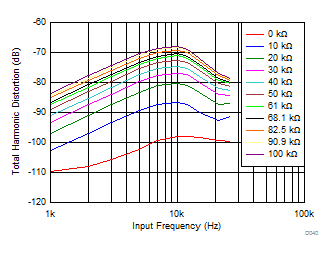Figure 44. THD vs Input Frequency for Different Source Impedances (±10 V)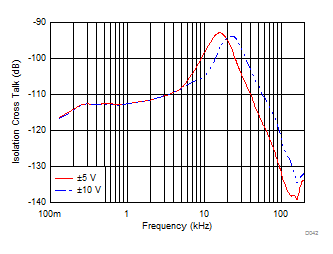Figure 46. Isolation Crosstalk vs Noise Frequency
(Inputs Within Range)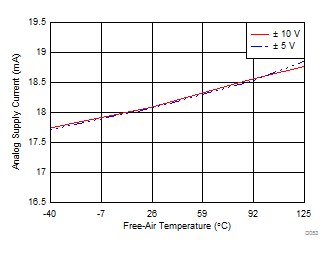Figure 48. Analog Supply Current (Operational) vs TemperatureFigure 50. Analog Supply Current vs Temperature
(Standby)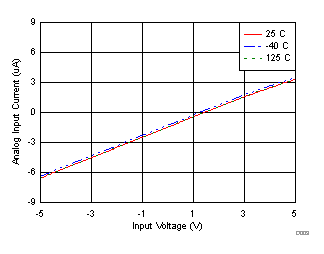Figure 11. Analog Input Current vs Input Voltage Over Temperature (±5 V)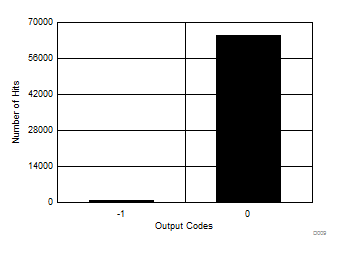Mean = –0.012, sigma = 0.11, number of hits = 65536, VIN = 0 V
Figure 13. DC Histogram of Codes (±10 V)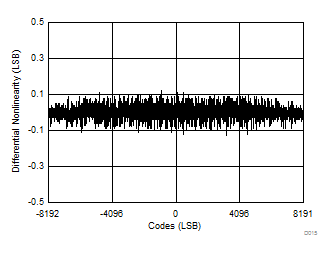Figure 15. DNL for All Codes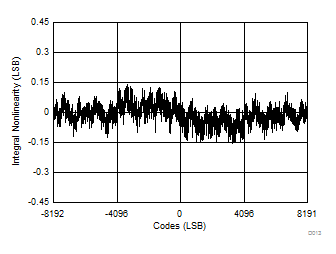Figure 17. INL vs All Codes (±10 V)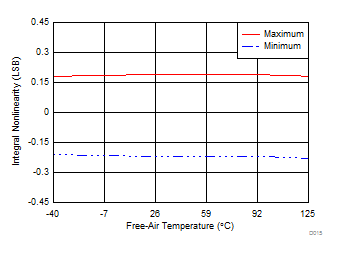Figure 19. INL vs Temperature (±10 V)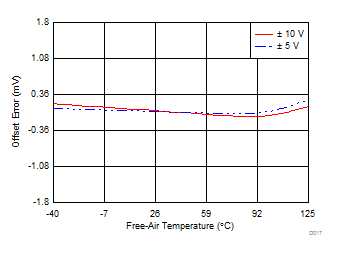Figure 21. Offset Error vs Temperature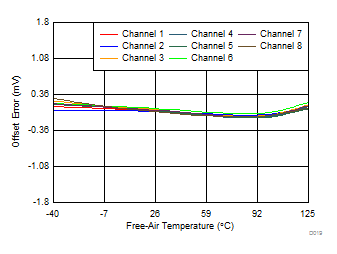Figure 23. Offset Error Across Channels vs Temperature
(±10 V)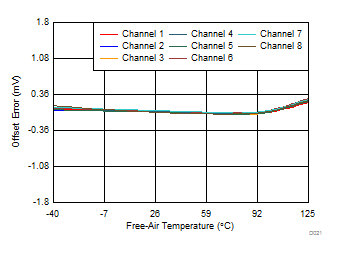Figure 25. Offset Error Across Channels vs Temperature
(±5 V)External reference
Figure 27. Gain Error Drift Histogram Distribution (±10 V)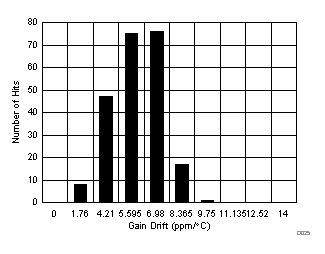External reference
Figure 29. Gain Error Drift Histogram Distribution (±5 V)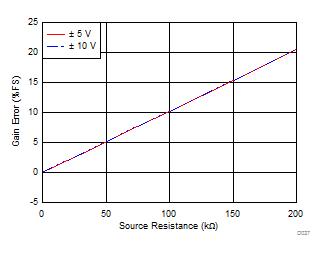Figure 31. Gain Error as a Function of External Source ResistanceNumber of points = 32k, SNR = 85.26 dB, SINAD = 85.23 dB, THD = –110.25 dB, SFDR = 114.08 dB
Figure 33. Typical FFT Plot (±5 V)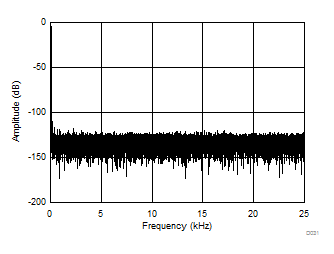Number of points = 32k, SNR = 85.42 dB, SINAD = 85.39 dB, THD = –110.6 dB, SFDR = 115.27 dB
Figure 35. Typical FFT Plot for OSR 4x (±5 V)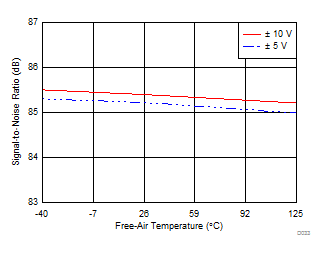OSR = 0
Figure 37. SNR vs Temperature for Different Input Ranges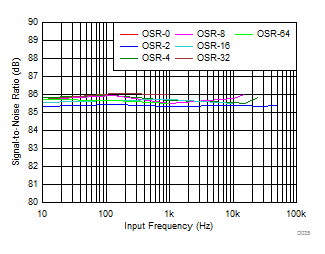Figure 39. SNR vs Input Frequency for Different OSR
(±5 V)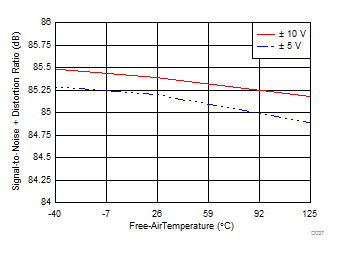OSR = 0
Figure 41. SINAD vs Temperature for Different Input Ranges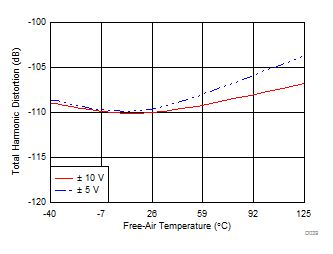Figure 43. THD vs Temperature for Different Input Ranges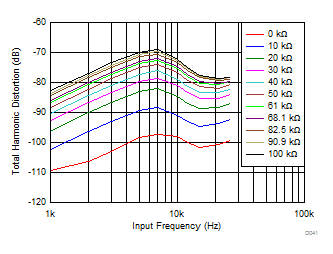Figure 45. THD vs Input Frequency for Different Source Impedances (±5 V)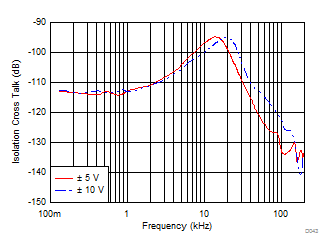Figure 47. Isolation Crosstalk vs Noise Frequency (Saturated Inputs)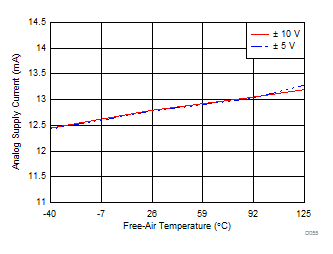Figure 49. Analog Supply Current (Static) vs Temperature (Sampling)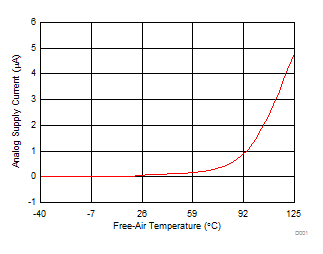Figure 51. Analog Supply Current vs Temperature (Shutdown)